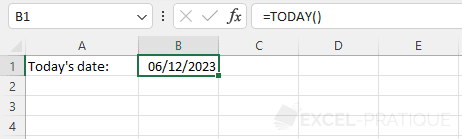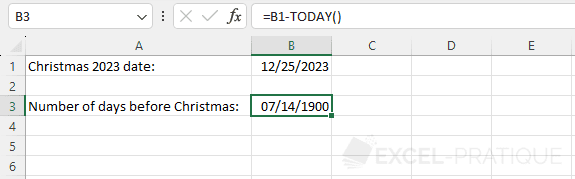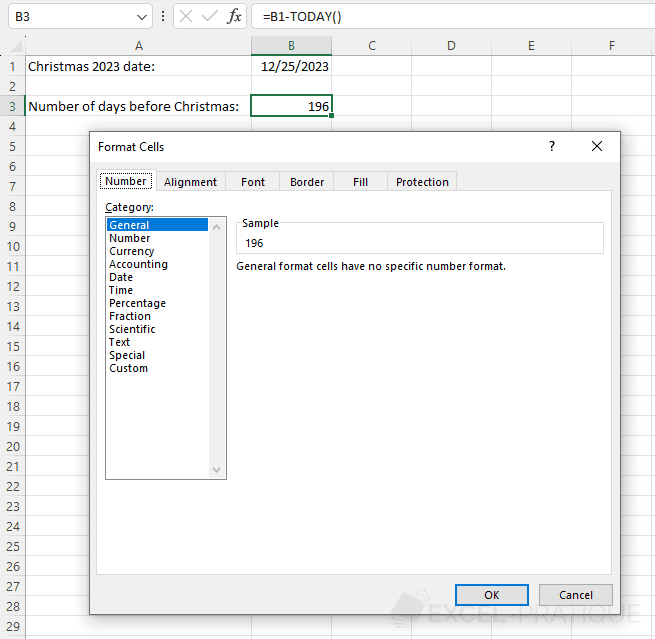# Excel Function: TODAY

The Excel function TODAY displays the current date (updated automatically every day).

This function can also be used to calculate the interval between the current date and a date of your choice.

Usage:

`=TODAY()`

## Examples of use

To get today's date, simply enter into a cell:

``=TODAY()``To calculate the number of days remaining until the date in cell B1, enter:

``=B1-TODAY()``To get a number of days instead of the date, change the formatting of cell B3:You will then get the remaining number of days which will be updated every day.